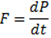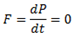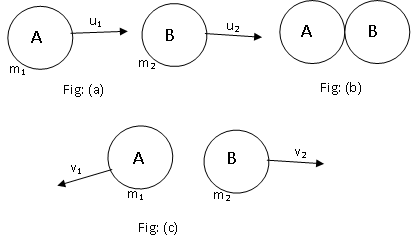## TyroCity

Physics XI Notes for Physics Notes

Posted on • Updated on

# Law Of Conservation Of Linear Momentum

The law of conservation of linear momentum states that if no external forces act on the system of two colliding objects, then the vector sum of the linear momentum of each body remains constant and is not affected by their mutual interaction.

Alternatively, it states that if net external force acting on a system is zero, the total momentum of the system remains constant.
Proof:
Let us consider a particle of mass ‘m’ and acceleration ‘a’. Then, from 2nd law of motion,If no external force acts on the body then, F=0,Therefore, ‘P’ is constant or conserved.
(Note: If the derivative of any quantity is zero, it must be a constant quantity.)

Deduction of law of conservation of linear momentum for two colliding bodiesLet us consider two bodies of masses m1 and m2 moving in straight line in the same direction with initial velocities u1 and u2. They collide for a short time ∆t. After collision, they move with velocities v1 and v2.
From 2nd law of motion,
Force applied by A on B = Rate of change of momentum of B
FAB = (m2v2-m2u2)/∆t
Similarly,
Force applied by B on A = Rate of change of momentum of A
FBA = (m1v1-m1u1)/∆t
From Newton’s 3rd law of motion,
FAB = -FBA
Or, (m2v2-m2u2)/∆t = -(m1v1-m1u1)/∆t
Or, m2v2-m2u2 = -m1v1+m1u1
Or, m1u1 + m2u2 = m1v1 + m2v2

This means the total momentum before collision is equal to total momentum after collision. This proves the law of conservation of linear momentum.